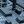# Silly RobotSuper Circuits wrote 03/14/2020 at 09:19 • 4 min read • Like

https://www.instructables.com/id/Waving-Robot/

After reading the article I found out that the two power transistors could still be ON even with the use of D1 and D2. This will cause a high power dissipation in the two transistors. Also, the user cannot raise the supply voltage above 3 V.

At the same article another circuit is shown with a voltage follower transistor configuration. Although the two transistors are not ON at the same time in second circuit, the voltage follower configuration does not allow full transistor saturation and cannot amplify the input signal. A Darlington transistor pair has the same problem.

Recently someone published the following MOSFET motor driver circuit on Hack A Day website:

Step 1: Design the Circuit

Thus I designed my own circuit with the use of PSpice simulation software:

You can see that "a" (Q2a and Q3a) and "b" (Q2b and Q3b) transistor pairs are completely isolated to ensure that they are not both ON at the same time. The Q1 transistor can also amplify small input signals. My circuit also does not require the use of big Cin bipolar capacitor (that was needed for the other circuit) that could be very expensive. The Cin capacitor is still needed to remove any DC voltages at the input of this circuit, unless you are connecting the directly circuit to 555 timer or transistor oscillator.

Calculate Rb3b resistor:

Assuming the C1 voltage is zero then the maximum current across the Rc3b resistor will be:

Ic3max = Vs / Rc3b = 3 V / 10 = 0.3 A

The base current will be 30 times smaller at worse case scenario:

Ib3max = Ic3max / Beta = 0.3 A / 30 = 10 mA

Rb3 = (Vs - Vbe) / Ib3max = (3 V - 0.7 V) / 10 mA

= 230 ohms or 220 ohms

I assume that the other transistors have a minimum current gain of just 20. I assumed a bigger current gain for the load Q3 transistors because I was hoping to use a higher Rb3 value to reduce the chances of burning the transistor base. A typical transistor gain is about 100 and some transistors can have a current gain as high as 500. Thus we have nothing to worry about.

Calculate Rb2 resistors:

Ib2max = Ic2max / Beta = 10 mA / 20 = 500 uA

0.84656884623

Rb2a = Rb2b =  (Vs - Vbe) / Ib2max = (3 V - 0.7 V) / 500 uA

= 2.3 V / 500 uA  = 4,600 ohms or 4.7 kohm

The transistor Q1a base current is equal to:

Ib1amax = Ic1max / Beta = ((Vs - Vbe) / Rb2b) / Beta

= (2.3 V / 4,700 ohms) / 20 = 0.0004893617 A / 20

= 24.4680851064 uA

Calculate Rb1 resistors:

Rb1c = Rb1a = (Vs - Vbe) / Ib1max = 2.3 V / 24.4680851064 uA

= 93999.99 ohms or 100 kohms

Calculate the lower high pass filter cut-off frequency:

fl = 1/(2*pi*Cin*Rb1) = 1/(2*pi*(4*470*10^-9)*100,000 ohms)

= 0.84656884623 Hz

This frequency is below the minimum of 10 Hz for audio amplifier. However, we are designing a moving robot. The rotation cycle frequency of a robot is usually well below 10 Hz. We can reduce the frequency by increasing the Cin capacitor value. However, that would mean that we need to use a bipolar capacitor that costs more money, this is why I added additional pillow 470 nF capacitors in parallel. Alternatively we can increase the Rb1a resistor value because I did mentioned before that a typical transistor current gain is usually well about 20 or even 30.

Step 2: Simulations

The simulations show that load current does not look very symmetrical:

The PSpice student edition software might not be accurate due to inaccurate transistor signal modelling of this software. This lack of accuracy is showing that one the power transistor will not fully turn off. In really life this might not be the case.

You might want to know if the Vc1 node can be connected directly to 555 timer, thus avoiding the Q1 transistor circuit. This is a hard question to answer because the 555 timer output must swing between 0 V and supply voltages. If the 555 timer output is similar op-amp that has a minimum 2 V saturation voltage limit than this circuit will not work. You can also try connecting a comparator (eg LM311) as an oscillator.

## Discussions